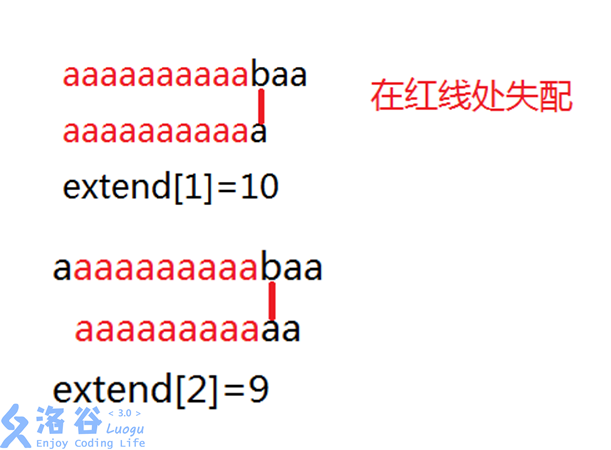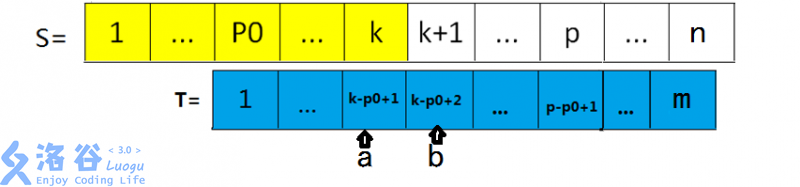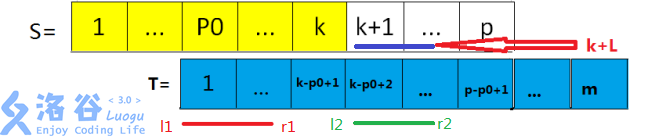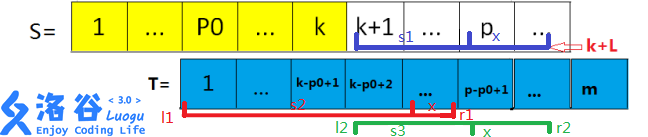# ez_lcw 的博客# ez_lcw 的博客

### 扩展kmp——神奇的字符串匹配

posted on 2019-03-15 14:02:15 | under 未分类 |

## 二、前置知识

1. kmp的算法思想，具体可以参考这篇日报

2. trie树（字典树）

## 三、经典扩展kmp模板问题：

$\mathcal{AC}$自动机？好像更不可做了。

## 例子：

$T=aaaaaaaaaaa$，$m=11$$∴S[2...10]=T[2...10]=T[1...9]$

## 对于一般情况：## 第一种情况：$k+L<p$if(i+nxt[i-p0]<extend[p0]+p0)extend[i]=nxt[i-p0];
//i相当于k+1
//nxt[i-p0]相当于L
//extend[p0]+p0相当于p
//因为在代码里我是从0开始记字符串的，所以本应在小于号左侧减1，现在不用了。

## 第二种情况：$k+L>=p$$T[l1...r1-x+1]=T[l2...r2-x+1]=S[k+1...(k+L)-x+1]$

int now=extend[p0]+p0-i;
now=max(now,0);//这里是防止i>p
while(t[now]==s[i+now]&&now<(int)t.size()&&now+i<(int)s.size())now++;//暴力求解的过程
extend[i]=now;
p0=i;//更新p0

## Code

#include<bits/stdc++.h>

#define N 1000010

using namespace std;

int q,nxt[N],extend[N];
string s,t;

void getnxt()
{
nxt=t.size();//nxt一定是T的长度
int now=0;
while(t[now]==t[1+now]&&now+1<(int)t.size())now++;//这就是从1开始暴力
nxt=now;
int p0=1;
for(int i=2;i<(int)t.size();i++)
{
if(i+nxt[i-p0]<nxt[p0]+p0)nxt[i]=nxt[i-p0];//第一种情况
else
{//第二种情况
int now=nxt[p0]+p0-i;
now=max(now,0);//这里是为了防止i>p的情况
while(t[now]==t[i+now]&&i+now<(int)t.size())now++;//暴力
nxt[i]=now;
p0=i;//更新p0
}
}
}

void exkmp()
{
getnxt();
int now=0;
while(s[now]==t[now]&&now<min((int)s.size(),(int)t.size()))now++;//暴力
extend=now;
int p0=0;
for(int i=1;i<(int)s.size();i++)
{
if(i+nxt[i-p0]<extend[p0]+p0)extend[i]=nxt[i-p0];//第一种情况
else
{//第二种情况
int now=extend[p0]+p0-i;
now=max(now,0);//这里是为了防止i>p的情况
while(t[now]==s[i+now]&&now<(int)t.size()&&now+i<(int)s.size())now++;//暴力
extend[i]=now;
p0=i;//更新p0
}
}
}

int main()
{
cin>>s>>t;
exkmp();
int len=t.size();
for(int i=0;i<len;i++)printf("%d ",nxt[i]);//输出nxt
puts("");
len=s.size();
for(int i=0;i<len;i++)printf("%d ",extend[i]);//输出extend
return 0;
}

$bzoj$和$hdu$上也有几道，大家可以去看看：

hdu2594 Simpsons’ Hidden Talents

hdu4333 Revolving Digits